# Space Themed Subtraction Puzzle

Puzzles are a great hands-on way to work on learning. The free space subtraction puzzle is great for working on subtraction for kids.

The hands-on puzzle is a great way to change up working on subtraction problems by giving them a fun puzzle to put together after they solve the equation.

This makes a great math center for kids working on subtraction and changes it up from worksheets and flashcards.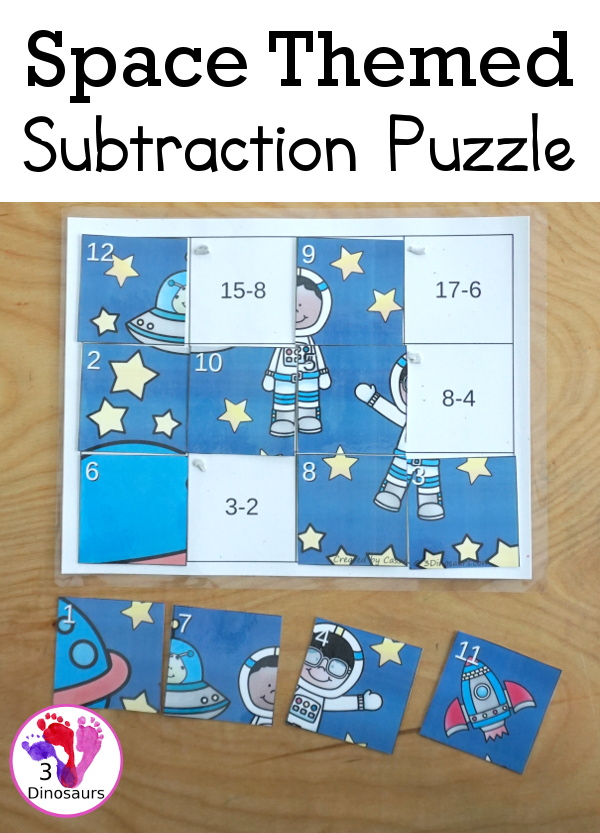We have included links to some of the products and resources we have used with this activity. If you purchase via the links we may earn a small commission.

This is a great addition to the space printables and subtraction printables on the site.

## What is Subtraction?

Subtraction is the removal of objects from a collection. So you start with 5 objects and take two of the objects away and you have three remaining.

The equation for subtraction would look like 5 – 2 = 3.

This subtraction puzzle works on the subtraction of numbers from 2 to 20.

The puzzle has very simple mat with equations on it. There are 12 subtraction equations that have answers from 1 t0 12. I recommend printing on cardstockand laminatingthe puzzles if you will use them often.

I will say to change things up I did use stardust cardstockfor our mat.Each piece of the puzzle has a number in the top left corner of each piece of the puzzle that will match a square on the mat. I printed the puzzle pieces on cardstockand laminatedit.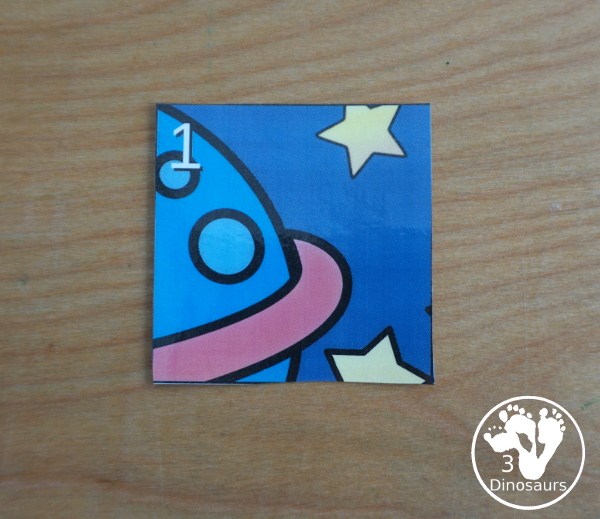## Things to use with the Space Subtraction Puzzle

These are the items we used with this subtractionpuzzle to make or prepare or activities the puzzle is apart of in the post.

• printer• white cardstock• stardust cardstock• laminator• laminating pouches• wall putty• Space TOOB
• sensory bin
• rice
• mini star erasers

## Ways to use the Space Subtraction Puzzle

One thing that I love to do with the puzzles in use wall putty on them. This makes the pieces of the puzzle stick in place. You can see I place a small amount of it on the corner. I like the putty because you can reuse it and I don’t need use something that is one-time use like velcro.Once put press the piece onto the putty it stays. You can see that I can hold up the puzzles and the pieces have not fallen off.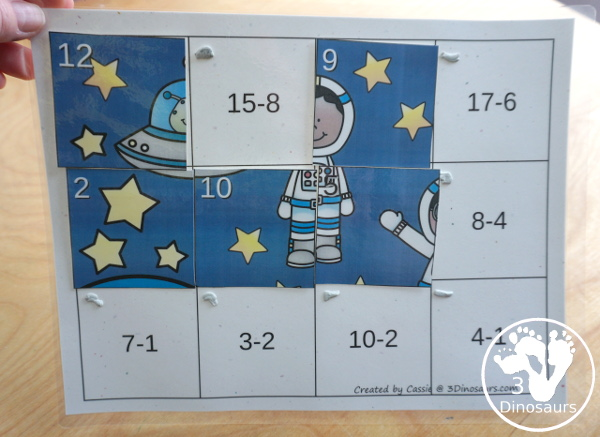One way to set up the puzzles is to have the puzzle and the cards right next to each other. This allows kids to do the puzzle.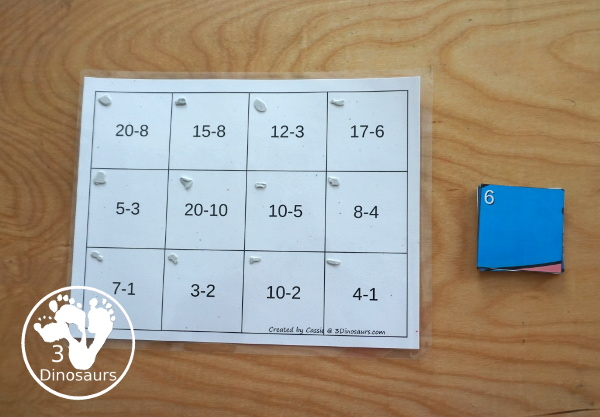They place each of the pieces of the puzzles on the mat as they solve the subtraction equations.If you want to change up the puzzle then put it in a sensory bin! We put rice, start erasers, the Space TOOB, and the puzzle pieces. It makes it is a sensory play as well as working on math.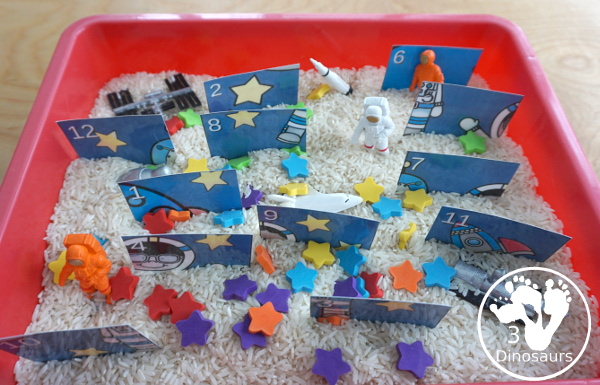They pull out the puzzle pieces and then find the matching equations on the mat.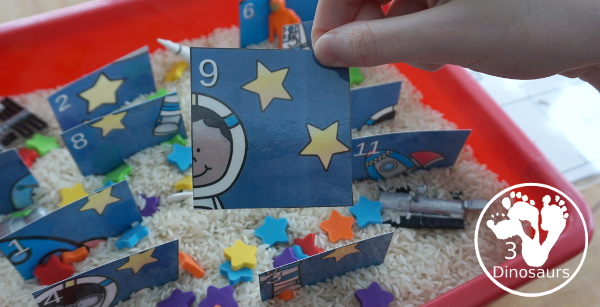## What you will find in the Free Space Subtraction Puzzle

• Subtraction from 20 to 1
• 12 piece puzzles
• Space theme for the puzzle
• 2 pages## Check out these other subtraction printables

Subtraction Flashcards: 1 to 10

Subtraction Bookmarks

Hands on Number Bond Mats: Addition & Subtraction

Check out all the fun Space Printables & Activities on 3 Dinosaurs.

Check out all the fun SubtractionPrintables & Activities on 3 Dinosaurs.

Cassie – 3Dinosaurs.com